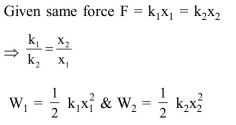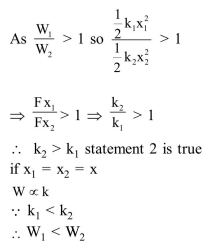Deepak Scored 45->99%ile with Bounce Back Crack Course. You can do it too!

# This question has Statement

Question:

This question has Statement-1 and Statement-2. of the four choices given after the statements, choose the one that best describes the two statements.

If two springs $\mathrm{S}_{1}$ and $\mathrm{S}_{2}$ of force constants $\mathrm{k}_{1}$ and $\mathrm{k}_{2}$, respectively, are stretched by the same force, it is found that more work is done on spring $S_{1}$ than on spring $S_{2}$.

Statement-1: If stretched by the same amount, work done on $\mathrm{S}_{1}$, will be more than that on $\mathrm{S}_{2}$ Statement-2 : $\mathrm{k}_{1}<\mathrm{k}_{2}$

1. Statement- 1 is true, Statement- 2 is true and Statement- 2 is not the correct explanation of Statement- 1 .

2. Statement-1 is false, Statement- 2 is true

3. Statement-1 is true, Statement- 2 is false

4. Statement-1 is true, Statement-2 is true and Statement-2 is the correct explanation of statement-1.

Correct Option: , 2

Solution: